# HiSET Mathematics: Circular Arcs and Circles Chapter Exam

Exam Instructions:

Choose your answers to the questions and click 'Next' to see the next set of questions. You can skip questions if you would like and come back to them later with the yellow "Go To First Skipped Question" button. When you have completed the practice exam, a green submit button will appear. Click it to see your results. Good luck!

### Page 1

#### Question 2 2. If the measure of central angle AOB is 62 degrees, what is the measure of inscribed angle ACB?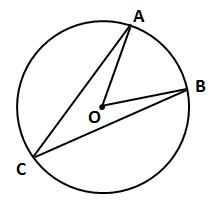#### Question 5 5. In the picture below, if arc CE is 172 degrees and arc BD is 80 degrees, what is the measure of angle CAE?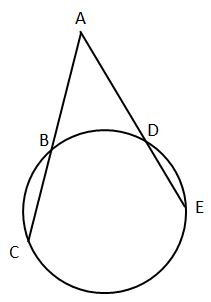### Page 2

#### Question 9 9. In the picture below, if arc BD is 168 degrees and arc BC is 70 degrees, what is the measure of angle BAD?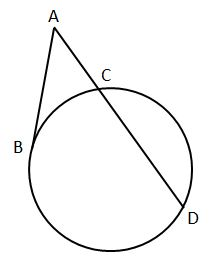#### Question 10 10. In the picture below, if major arc BC is 258 degrees and minor arc BC is 102 degrees, what is the measure of angle BAC?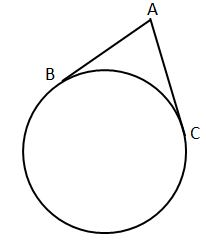### Page 3

#### Question 13 13. If arc AC is 122 degrees and arc BC is 192 degrees, what is the measure of angle ACB?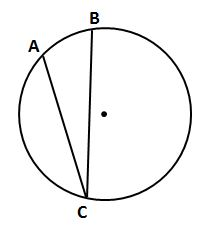#### Question 14 14. In the picture below, if arc AC is 146 degrees and arc BD is 32 degrees, what is the measure of angle AEC?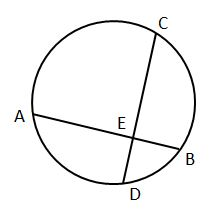#### Question 15 15. In the picture below, if AB is 7, BC is 19 and AD is 8, what is the length of DE?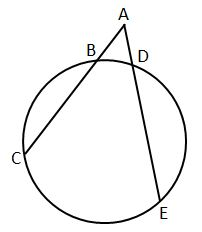### Page 4

#### Question 16 16. In the picture below, if AE is 10, AB is 14 and ED is 16, what is the length of CE?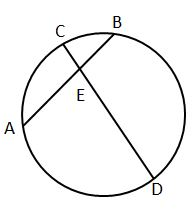#### Question 17 17. If arc BC is 84 degrees, what is the measure of angle ACB?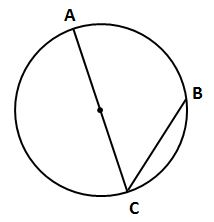#### Question 20 20. If angle ACB is 34 degrees, what is the measure of angle ADB?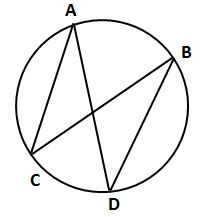### Page 5

#### Question 21 21. If angle ACB is 47 degrees, what is the measure of angle AOB?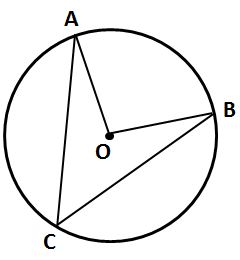#### Question 23 23. In the pictured circle, the measure of angle ACB is 22 degrees and the measure of angle BXD is 120 degrees. What is the measure of angle CAD?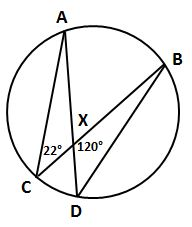#### Question 24 24. In the picture below, if arc AB is 82 degrees, what is the measure of angle BAC?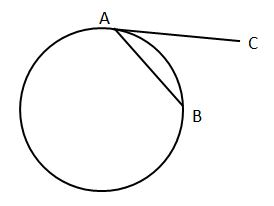#### Question 25 25. If arc AB is 72 degrees, what is the measure of angle ACB?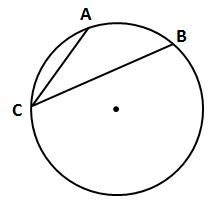### Page 6

#### Question 27 27. If arc AC is 106 degrees and arc BC is 82 degrees, what is the measure of angle ACB?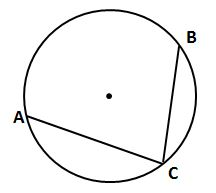#### Question 30 30. If angle AOB is 40 degrees, what is the measure of angle ACB?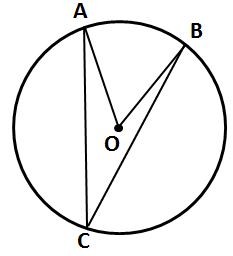#### HiSET Mathematics: Circular Arcs and Circles Chapter Exam Instructions

Choose your answers to the questions and click 'Next' to see the next set of questions. You can skip questions if you would like and come back to them later with the yellow "Go To First Skipped Question" button. When you have completed the practice exam, a green submit button will appear. Click it to see your results. Good luck!

Support